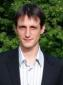# Longitudinal studies and categorical latent variable

6 posts / 0 newOffline
Joined: 10/13/2009 - 05:02
Longitudinal studies and categorical latent variable

Will Open Mx be able to deal with such problem.I am in the begining of a PHD and I apm looking for a software to test such problem, being familiar with R and on mac os X I was wondering if OpenMx was an option ?Thank you for this work.Guillaume AveninOffline
Joined: 07/31/2009 - 05:40
Categorical latent variable

Categorical latent variable modeling is in the works.  Two of our core developers have a plan and are currently working on it, and I'm sure they would appreciate testing when the time comes, if you were interested. With regards to longitudinal studies, as long as your two interests are mutually exclusive, you should be able to model longitudinal data in OpenMx using standard techniques.  But, if you are interested in GMMs or LTA, then my first comment applies.Offline
Joined: 10/13/2009 - 05:02

Thank you for this answer, your first comment answered precisely to my interogation and I would be very glad to be some help.Actually I will pursue the exploration of this beautifull software (R extension), and hope be able to model longitudinal data with continuous variables also.Offline
Joined: 11/28/2013 - 06:10
Hi Jeff,I have two questions

Hi Jeff,

I have two questions concerning latent class analysis (LCA) and latent transition analysis (LTA), respectively.

1. I followed the LCA example code from here: http://www.vipbg.vcu.edu/NIDAworkshop2012/LCA_example_4c.R
and tried to replicate the results of ex7.3 on Mplus 7 manual(without auxiliary statement). The class membership probabilities for each latent class were consistent between OpenMx and Mplus, however, I noticed that the number of parameter that OpenMx and Mplus estimated were different, 10 vs. 9. A closer look at the results showed that the parameters the two packages estimated are:

OpenMx: 8 thresholds of indicators+2 class membership probabilities=10 parameters.
Mplus: 8 thresholds of indicators+1 mean of c#1=9 parameters
(Mplus also estimated the 2 class membership probabilities, but these 2 seems not in the 9 parameters).

So how can I estimate the mean of c#1? Besides, the standard error I got for the estimates are NA. How to get the standard error? and is it possible to include auxiliary variables in openmx?

1. I saw information here and there online that openmx is capable of doing LTA. However, I could not find any example about it. I am trying to replicate the results of ex8.13 and ex8.14 of Mplus 7 manual. Could you give me some suggestions please?

Here is what I have so far (the model did not converge):

> c11=mxModel('c11',
+ mxMatrix(type='Iden',name='r',nrow=5,ncol=5,byrow=T,free=F),
+ mxMatrix(type='Full',name='m',nrow=1,ncol=5,byrow=T,free=F),
+ mxMatrix(type='Full',name='c1thrshd',nrow=1,ncol=5,byrow=T,free=T),
+ )
> c12=mxModel('c12',
+ mxMatrix(type='Iden',name='r',nrow=5,ncol=5,byrow=T,free=F),
+ mxMatrix(type='Full',name='m',nrow=1,ncol=5,byrow=T,free=F),
+ mxMatrix(type='Full',name='c2thrshd',nrow=1,ncol=5,byrow=T,free=T),
+ )
> c13=mxModel('c13',
+ mxMatrix(type='Iden',name='r',nrow=5,ncol=5,byrow=T,free=F),
+ mxMatrix(type='Full',name='m',nrow=1,ncol=5,byrow=T,free=F),
+ mxMatrix(type='Full',name='c3thrshd',nrow=1,ncol=5,byrow=T,free=T),
+ )
> c21=mxModel('c21',
+ mxMatrix(type='Iden',name='r',nrow=5,ncol=5,byrow=T,free=F),
+ mxMatrix(type='Full',name='m',nrow=1,ncol=5,byrow=T,free=F),
+ mxMatrix(type='Full',name='c1thrshd',nrow=1,ncol=5,byrow=T,free=T),
+ )
> c22=mxModel('c22',
+ mxMatrix(type='Iden',name='r',nrow=5,ncol=5,byrow=T,free=F),
+ mxMatrix(type='Full',name='m',nrow=1,ncol=5,byrow=T,free=F),
+ mxMatrix(type='Full',name='c2thrshd',nrow=1,ncol=5,byrow=T,free=T),
+ )
> c23=mxModel('c23',
+ mxMatrix(type='Iden',name='r',nrow=5,ncol=5,byrow=T,free=F),
+ mxMatrix(type='Full',name='m',nrow=1,ncol=5,byrow=T,free=F),
+ mxMatrix(type='Full',name='c3thrshd',nrow=1,ncol=5,byrow=T,free=T),
+ )
> c1toc2=mxModel('c1toc2trans',
+ mxMatrix(type='Full',name='tr',nrow=3,ncol=3,values=c(1,1,1,1,1,1,1,1,1),byrow=T,free=T)
+ )
+ mxMatrix('Full',name='c1memprob',nrow=3,ncol=1,byrow=T,free=T,labels=c('c11prob','c12prob','c13prob')),
+ mxMatrix('Full',name='c2memprob',nrow=3,ncol=1,byrow=T,free=T,labels=c('c21prob','c22prob','c23prob')),
+ mxBounds(c('c11prob','c12prob','c13prob','c21prob','c22prob','c23prob'),0,1),
+ mxAlgebra(sum(c1memprob),name='c1p'),
+ mxAlgebra(sum(c2memprob),name='c2p'),
+ mxConstraint(c1p==1),
+ mxConstraint(c2p==1),
+ mxAlgebra(-2*sum(log(c11prob%x%c11.objective+c12prob%x%c12.objective+c13prob%x%c13.objective+c21prob%x%c21.objective+c22prob%x%c22.objective+c23prob%x%c23.objective)),name='lta'),
+ mxAlgebraObjective('lta')
+ )
> model=mxRun(ltamodel)
Running ltamodel
Warning message:
In model 'ltamodel' NPSOL returned a non-zero status code 1. The final iterate satisfies the optimality conditions to the accuracy requested, but the sequence of iterates has not yet converged. NPSOL was terminated because no further improvement could be made in the merit function (Mx status GREEN).
> summary(model)

Thank you very much.Offline
Joined: 07/31/2009 - 15:14
May work but

What you have may work but it isn't very efficient. This is because OpenMx will use multidimensional integration over 5 dimensions, but the covariance matrix is an identity matrix. Classic Mx would detect such independence, but OpenMx does not yet do that.

I'm not an Mplus expert, but from looking at the manual online it seems that there is another variable in the dataset which gives an observed class for two groups. The model seems to specify that the groups differ with respect to the mean of the latent variable. In OpenMx this could be achieved by a definition variable for group, read in with the data (like x in the Mplus script) or by a multiple group approach. I am thinking that the group variable might be simpler - code x 0/1 and then put in a group mean algebra of the form:

mxMatrix(type='Full',name='x',nrow=1,ncol=1,labels='data.x', free=F),
mxMatrix(type='Full',name='groupMean',nrow=1,ncol=5,labels='mu_x', free=T),
mxAlgebra(x %x% groupMean, name="mAlgebra") 

and then change

mxFIMLObjective(covariance='r',means='m',thresholds='c3thrshd',dimnames=names(data[,6:10]),vector=T)

to

mxFIMLObjective(covariance='r',means='mAlgebra',thresholds='c3thrshd',dimnames=names(data[,6:10]),vector=T)

To get the standard errors, you probably have to get rid of any mxConstraint being used. This is pretty easy for class membership probabilities, one can simply fix one of them to 1.0 and then make an algebra to divide them by their sum, along these lines:

initialProbs <- mxAlgebra(rawInitialProbs %x% (1/sum(rawInitialProbs)), name="initialProbs")

I have a regime switching growth curve model paper submitted to Psych Methods, which probably does quite a bit of what you want. I could share a draft with you if that would help.Offline
Joined: 11/28/2013 - 06:10
Dear Neale,Thank you very

Dear Neale,

Thank you very much for replying.

You are correct. The openmx code I have now does not include the binary covariate. I was in the first step of trying, just want to see if the model works (apparently something went wrong).

Could you share the draft and maybe some example code for the regime switching model please? That would be very helpful.

Thank you very much